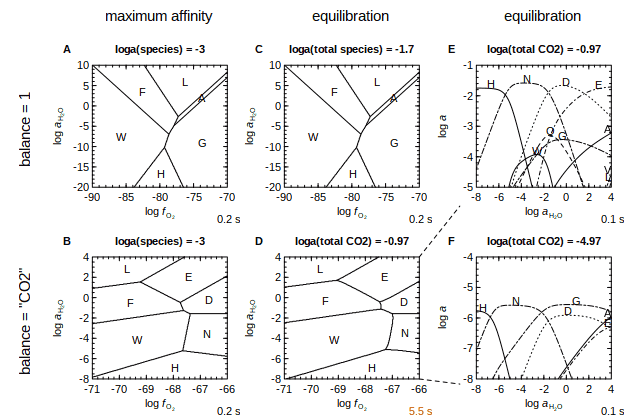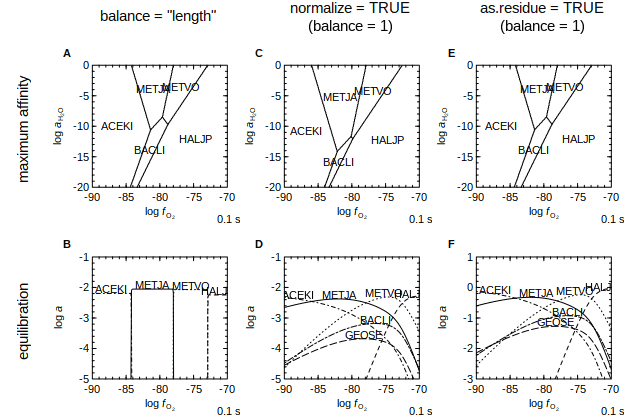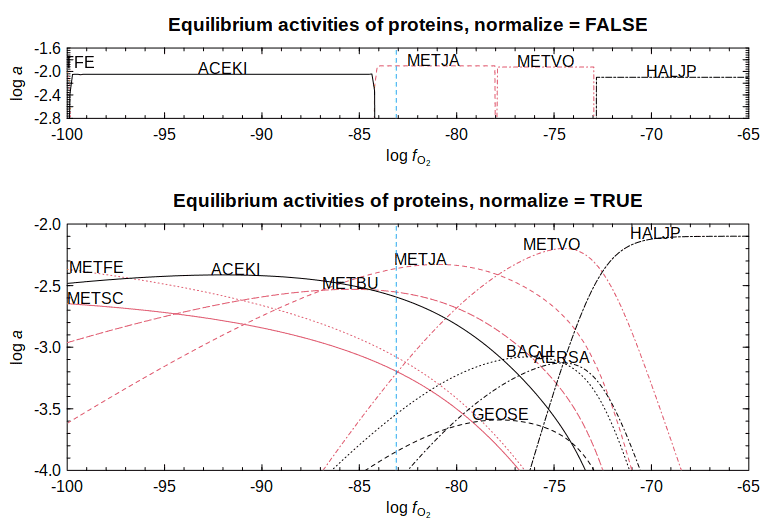# Equilibrium in CHNOSZ

This vignette was compiled on 2021-04-08 with CHNOSZ version 1.4.1. This document defines the concepts and provides examples of equilibrium calculations in CHNOSZ.

First, some limitations. CHNOSZ is not a general-purpose speciation code. The types of equilibrium calculations it can handle are restricted to those systems that can be described as balanced on an element. For example, we can predict the speciation of Au in complexes with chloride, hydroxide, and sulfide all with specified activities. But we can not at the same time predict the formation of species such as NaCl(aq), which is required for a complete equilibrium model.

## Concepts

System

Chemical system defined by formed species (referred to simply as species) and basis species, which are analogous to thermodynamic components. The possible species are any that are available in the OBIGT thermodynamic database.

Species
Selection of possible chemical species for which you want to calculate relative stabilities.
Basis species
Any minimal set of possible species that can be used to balance the formation reactions of the species. Additionally, in CHNOSZ the number of basis species must be equal to the number of elements.
Formation reaction
Chemical reaction giving the stoichiometry of basis species combined to make 1 mole of a species.
Transformation reaction
Any combination of two formation reactions in opposite directions (1 mole of a species reacts to form 1 mole of another species).
Balancing coefficients
(nbalance) For a system that is balanced on one of the basis species, the number of moles of that basis species in the formation reaction of each of the species. The number can be positive or negative but not zero. Can also represent a virtual quantity, such as 1 (balance on moles of species) or number of amino acids in a protein sequence (balance on protein length).
Speciation diagram
Diagram showing the equilibrium activities of species as a function of one variable.
Predominance diagram
Diagram variables showing fields for species with highest equilibrium activity as a function of two variables. Also known as an equal activity diagram for aqueous species or stability diagram for minerals.
Chemical affinity
Negative of the differential of Gibbs energy of a system with respect to reaction progress. For a given reaction, chemical affinity is the negative of Gibbs energy of reaction: A = 2.303RTlog(K/Q), where K is the equilibrium constant and Q is the activity quotient of species in the reaction (log here denotes base-10 logarithms, i.e. `log10` in R).
Maximum affinity method
Approach used to construct predominance diagrams not by finding the highest activity at equilibrium but by finding the highest affinity at a reference activity. The reference activities are user-defined equal activities of species (e.g. unit activity for minerals and 10-3 for aqueous species).
Equilibration method

Calculations of the activities of species in equilibrium.

Metastable equilibrium
The condition that the affinities of all transformation reactions are zero. Implemented with the `equilibrate()` function in CHNOSZ, which is used below.
Total equilibrium
The condition that the affinities of all formation reactions are zero. Implemented with the `solubility()` function in CHNOSZ, which is not described here.

## Example 1: Amino acids

In this sytem the basis species are CO2, H2O, NH3, H2S, and O2 and the formed species are 20 amino acids.

``````library(CHNOSZ)
reset()
basis("CHNOS")
species(aminoacids(""))``````

These functions are used for the different diagrams in the figure.

``````aaA <- function() {
a <- affinity(O2 = c(-90, -70), H2O = c(-20, 10))
diagram(a, balance = 1, names = aa)
}

aaB <- function() {
a <- affinity(O2 = c(-90, -70), H2O = c(-20, 10))
e <- equilibrate(a, balance = 1)
diagram(e, names = aa)
}

aaC <- function() {
a <- affinity(O2 = c(-71, -66), H2O = c(-8, 4))
diagram(a, balance = "CO2", names = aa)
}

a <- affinity(O2 = c(-71, -66), H2O = c(-8, 4))
e <- equilibrate(a, balance = "CO2")
diagram(e, names = aa)
}

aaE <- function() {
basis("O2", -66)
a <- affinity(H2O = c(-8, 4))
e <- equilibrate(a, balance = "CO2")
diagram(e, ylim = c(-5, -1), names = aa)
}

aaF <- function() {
species(1:20, -7)
a <- affinity(H2O = c(-8, 4))
e <- equilibrate(a, balance = "CO2")
diagram(e, ylim = c(-8, -4), names = aa)
}``````

The labels used for the diagrams are the one-letter abbreviations for the amino acids.

``````aa <- aminoacids()
aa``````
``````##   "A" "C" "D" "E" "F" "G" "H" "I" "K" "L" "M" "N" "P" "Q" "R" "S" "T" "V" "W"
##  "Y"``````

Press the button to show all of the code for making the figure.Diagrams A and B use the maximum affinity method, where the reference activities of species are set to a single value. Diagrams C and D use the equilibration method, where the activities of species change across the diagram and the lines are drawn at equal activity. Other comments on the figure:

• The equal-activity lines in Diagrams A and C are the same. That is, with the setting `balance = 1`, the conditions for equal activity of two species do not depend on the actual value of that activity.

• Diagrams B and D are different. Because balance ≠ 1 (in this case, the reactions are balanced on CO2), the conditions for equal activity of species depend on the actual value of that activity. In particular, with the equilibration method, the lines are curved due to the distribution of more than two species.

• Diagrams E and F both use the equilibration method to calculate activities of species as a function of logaH2O at logfO2 = -66. Diagram F shows the default setting, where aCO2 is taken from the sum of initial activities of species (each 10-3). The positions of equal activities (where the lines cross) are the same as in Diagram D at logfO2 = -66.

• Diagram F is drawn for a lower total activity of aCO2. Not only are the activities of all amino acids decreased, but glycine starts to become predominant at some conditions. This is because, compared to other amino acids, it is smaller and has a lower coefficient on CO2 in its formation reaction.

## Example 2: Proteins

In this sytem the basis species are CO2, H2O, NH3, H2S, O2, and H+ and the formed species are 6 proteins from the set of archaeal and bacterial surface layer proteins considered by Dick (2008).

The inclusion of charge in the basis species (with H+) allows ionization of proteins to be calculated (Dick et al., 2006).

``````basis("CHNOS+")
organisms <- c("METJA", "HALJP", "METVO", "ACEKI", "GEOSE", "BACLI")
proteins <- c(rep("CSG", 3), rep("SLAP", 3))
species(proteins, organisms)``````

Here are the lengths of the proteins.

``protein.length(species()\$name)``
``##   530  828  553  736 1198  844``

These functions are used for the different diagrams in the figure.

``````prA <- function() {
a <- affinity(O2 = c(-90, -70), H2O = c(-20, 0))
diagram(a, balance = "length", names = organisms)
}

prB <- function() {
a <- affinity(O2 = c(-90, -70))
e <- equilibrate(a, balance = "length")
diagram(e, names = organisms, ylim = c(-5, -1))
}

prC <- function() {
a <- affinity(O2 = c(-90, -70), H2O = c(-20, 0))
diagram(a, normalize = TRUE, names = organisms)
}

prD <- function() {
a <- affinity(O2 = c(-90, -70))
e <- equilibrate(a, normalize = TRUE)
diagram(e, names = organisms, ylim = c(-5, -1))
}

prE <- function() {
a <- affinity(O2 = c(-90, -70), H2O = c(-20, 0))
diagram(a, as.residue = TRUE, names = organisms)
}

prF <- function() {
a <- affinity(O2 = c(-90, -70))
e <- equilibrate(a, as.residue = TRUE, loga.balance = 0)
diagram(e, names = organisms, ylim = c(-3, 1))
}``````Diagrams A, C, and E are predominance (equal-activity) diagrams made with different balancing constraints. Diagrams B, D, and F show a cross-section of the same system at logaH2O = 0.

• In Diagrams A and B, the reactions are balanced on protein length. The drastic transitions between proteins in B result from the large balancing coefficients, which become exponents on activities in the expression for the activity quotient (Q).

• In Diagrams C and D, the chemical formulas of the proteins are normalized, or divided by the protein length, before the equilibration or maximum affinity calculation. However, the final diagram is not drawn for activities of these “residue equivalents”, but for rescaled activities of whole proteins. Because of the rescaling, the fields of the shorter METJA and METVO proteins grow at the expense of the longer BACLI protein.

• Diagrams E and F are like the normalization calculation, except that the final diagram is drawn for the residue equivalents and not the whole proteins. The predominance diagram is the same as that for non-normalized reactions (A), but the equilibrium activities of residues are higher than those of the proteins.

## Example 3: Normalization

Here is another figure showing the effects of normalization. This is like Figure 5 of Dick (2008), extended to more extreme conditions. If you wish to reproduce the diagram from the 2008 paper more closely, uncomment the `add.OBIGT()` command.

``````#add.OBIGT("OldAA")
organisms <- c("METSC", "METJA", "METFE",  "METVO", "METBU",
"HALJP", "ACEKI", "GEOSE", "BACLI", "AERSA")
proteins <- c(rep("CSG", 6), rep("SLAP", 4))
# use red for Methano* genera
col <- c(rep(2, 5), rep(1, 5))
basis("CHNOS+")
species(proteins, organisms)
a <- affinity(O2 = c(-100, -65))
layout(matrix(1:2), heights = c(1, 2))
e <- equilibrate(a)
diagram(e, ylim = c(-2.8, -1.6), names = organisms, col = col)
water.lines(e, col = 4)
title(main="Equilibrium activities of proteins, normalize = FALSE")
e <- equilibrate(a, normalize = TRUE)
diagram(e, ylim = c(-4, -2), names = organisms, col = col)
water.lines(e, col = 4)
title(main = "Equilibrium activities of proteins, normalize = TRUE")``````Although it is below the stability limit of H2O (vertical dashed line), there is an interesting convergence of the metastable equilibrium activities of some proteins at low log fO2.

## Document history

• 2009-11-29 Initial version with CSG example, titled “Calculating relative abundances of proteins”
• 2012-09-30 Renamed to “Equilibrium in CHNOSZ”; remove activity comparisons and add maximum affinity method.
• 2015-11-08 Move previous material to Appendix and add sections on concepts, organization, examples, and applications; use knitr vignette engine.
• 2020-07-10 Simplify to concepts and examples (amino acids, proteins, normalization); convert document from LaTeX (Rnw) to R Markdown (Rmd).